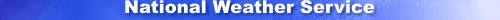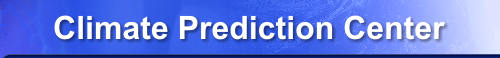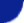Site Map News Organization SearchHOME > Monitoring_and_Data > Oceanic and Atmospheric Data > Reanalysis: Atmospheric Data > wgrib2-set_prob

Introduction

The -set_prob option converts some common non-probability Product Definition Template (PDT) to a probability PDT. Then it adds the metadata for the probability part of the PDT.

Step 1:
If current PDT is 0,1,2,3,4 or 6, then the PDT is converted to 6 (4.6)
using the -set_pdt +5 option.

If current PDT is 8,10,11,12,13,14 or 15, the he PDT is converted to 9 (4.9)
using the -set_pdt +9 option.

Step 2:
If the current PDT does not have Code Table 4.9 entry, print a warning
and return.

Step 3:
Fill in the various probability metadata using arguments to the option.

X=Forecast probability number
Y=Total number of forecast probabilities
Z=Probability Type (see Code Table 4.9)
A=lower limit
B=upper limit

Usage

-set_prob X Y Z A B
where X, Y, Z, A and B are defined above
for wgrib2 v3.0.0+, X, Y, Z, A or B can have the value "".
When the value "" is used, the previous value is not changed.

Example

\$ wgrib2 test2.grb
1:0:d=2009060500:TMP:2 m above ground:180 hour fcst:ENS=+19
\$ wgrib2 -set_prob 10 20 2 10.1 10.4  test2.grb
1:0:d=2009060500:TMP:2 m above ground:180 hour fcst:prob >=10.1 <10.4

X=10 Forecast probabilty number
Y=20 Total number of forecat probabilities (Number of intervals for probability forecasts?)
Z=2  Code Table 4.9
A=10 Lower limit (may not be used depending on Code Table 4.9)
B=10 Upper limit (may not be used depending on Code Table 4.9)